Ex 10.3

Chapter 10 Class 12 Vector Algebra
Serial order wise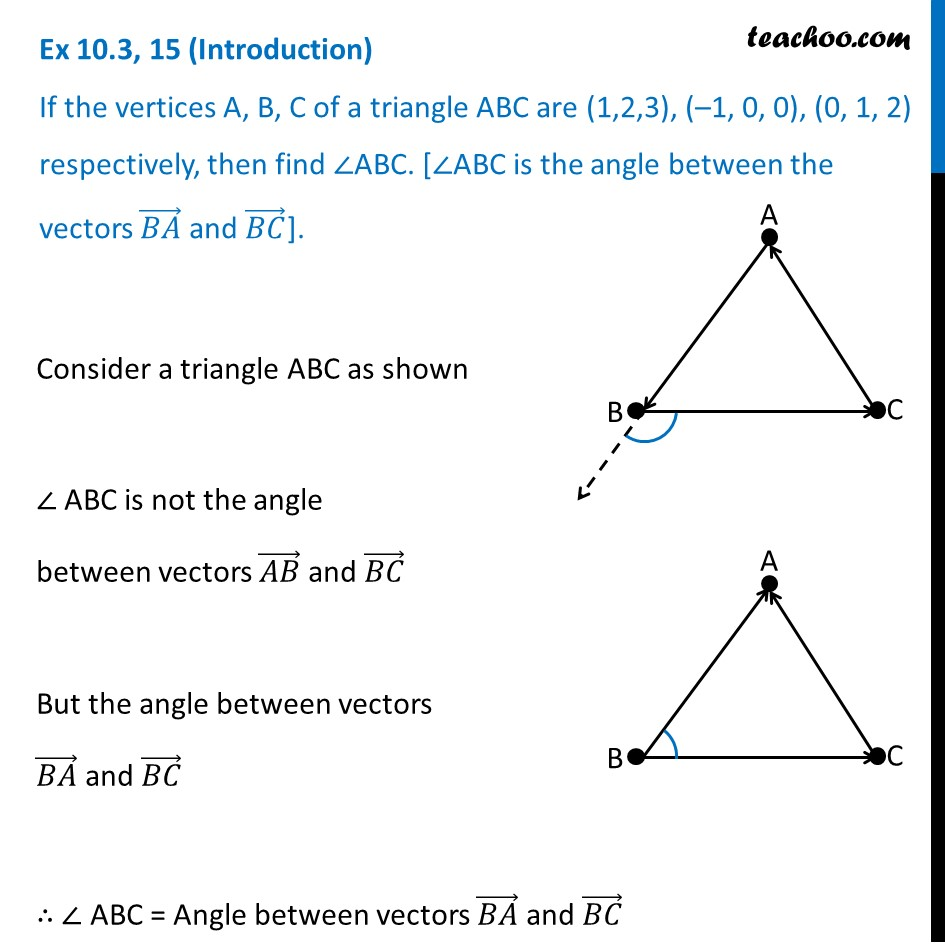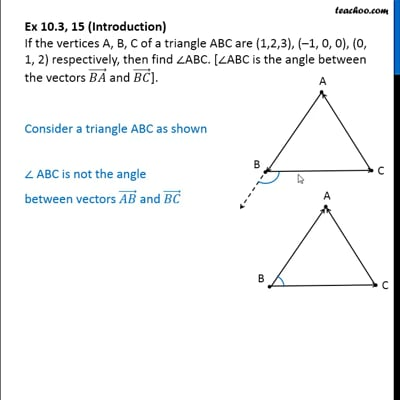This video is only available for Teachoo black users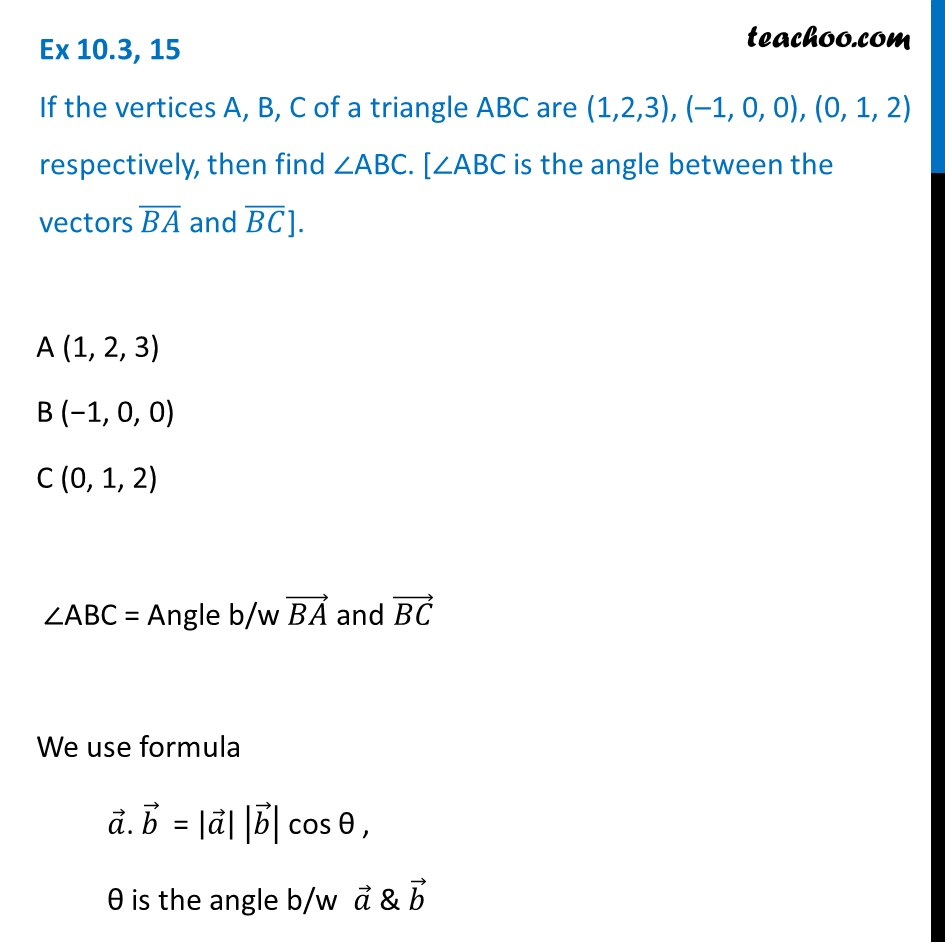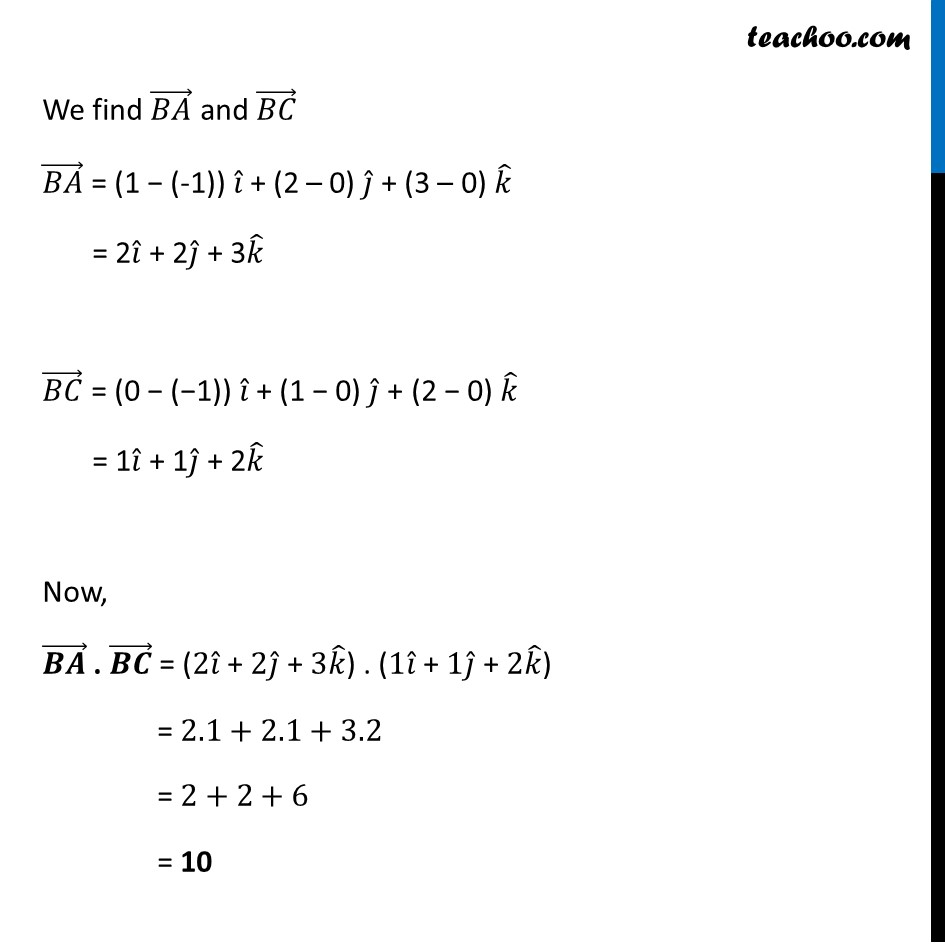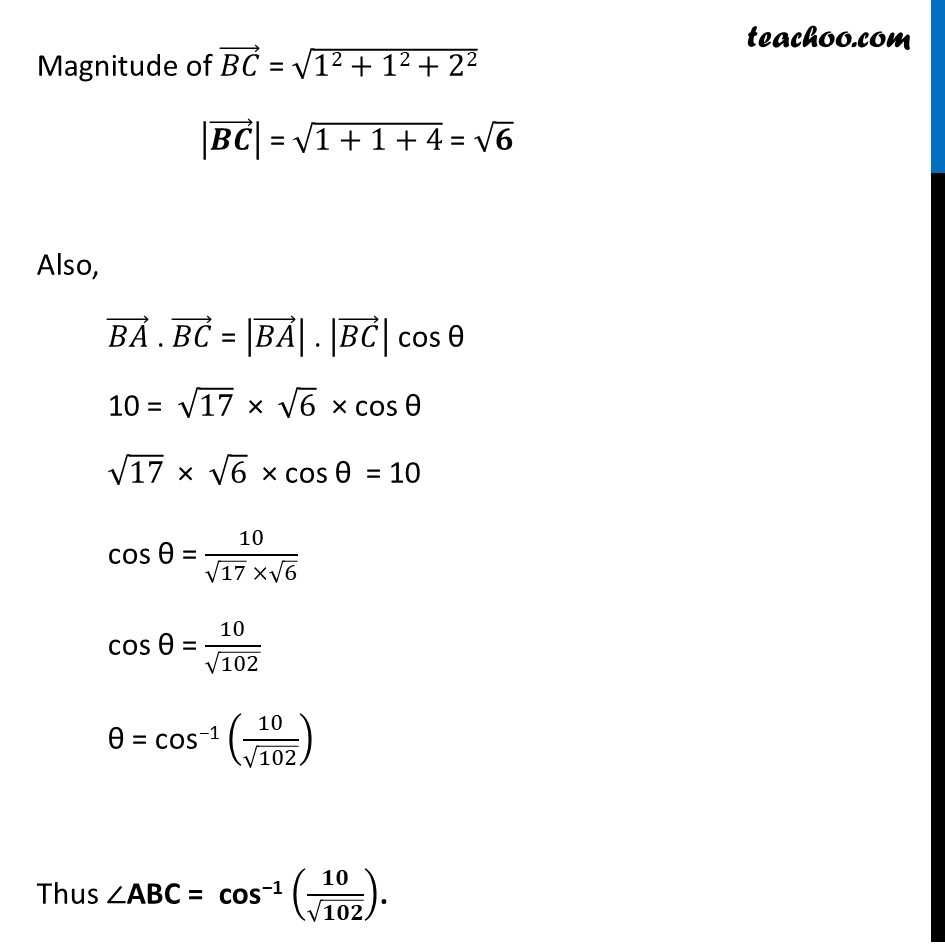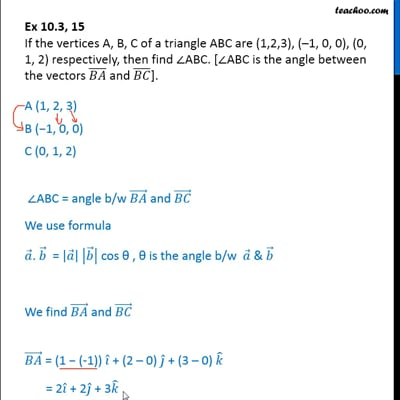This video is only available for Teachoo black users

Solve all your doubts with Teachoo Black (new monthly pack available now!)

### Transcript

Ex 10.3, 15 (Introduction) If the vertices A, B, C of a triangle ABC are (1,2,3), (–1, 0, 0), (0, 1, 2) respectively, then find ∠ABC. [∠ABC is the angle between the vectors (𝐵𝐴) ⃗ and (𝐵𝐶) ⃗]. Consider a triangle ABC as shown ∠ ABC is not the angle between vectors (𝐴𝐵) ⃗ and (𝐵𝐶) ⃗ But the angle between vectors (𝐵𝐴) ⃗ and (𝐵𝐶) ⃗ ∴ ∠ ABC = Angle between vectors (𝐵𝐴) ⃗ and (𝐵𝐶) ⃗ Ex 10.3, 15 If the vertices A, B, C of a triangle ABC are (1,2,3), (–1, 0, 0), (0, 1, 2) respectively, then find ∠ABC. [∠ABC is the angle between the vectors (𝐵𝐴) ̅ and (𝐵𝐶) ̅]. A (1, 2, 3) B (−1, 0, 0) C (0, 1, 2) ∠ABC = Angle b/w (𝐵𝐴) ⃗ and (𝐵𝐶) ⃗ We use formula 𝑎 ⃗. 𝑏 ⃗ = |𝑎 ⃗ | |𝑏 ⃗ | cos θ , θ is the angle b/w 𝑎 ⃗ & 𝑏 ⃗ We find (𝐵𝐴) ⃗ and (𝐵𝐶) ⃗ (𝐵𝐴) ⃗ = (1 − (-1)) 𝑖 ̂ + (2 – 0) 𝑗 ̂ + (3 – 0) 𝑘 ̂ = 2𝑖 ̂ + 2𝑗 ̂ + 3𝑘 ̂ (𝐵𝐶) ⃗ = (0 − (−1)) 𝑖 ̂ + (1 − 0) 𝑗 ̂ + (2 − 0) 𝑘 ̂ = 1𝑖 ̂ + 1𝑗 ̂ + 2𝑘 ̂ Now, (𝑩𝑨) ⃗ . (𝑩𝑪) ⃗ = ("2" 𝑖 ̂" + " 2𝑗 ̂" + " 3𝑘 ̂) . ("1" 𝑖 ̂" + " 1𝑗 ̂" + " 2𝑘 ̂) = 2.1+2.1+3.2 = 2+2+6 = 10 Magnitude of (𝐵𝐶) ⃗ = √(12+12+22) |(𝑩𝑪) ⃗ | = √(1+1+4) = √𝟔 Also, (𝐵𝐴) ⃗ . (𝐵𝐶) ⃗ = |(𝐵𝐴) ⃗ | . |(𝐵𝐶) ⃗ | cos θ 10 = √17 × √6 × cos θ √17 × √6 × cos θ = 10 cos θ = 10/(√17 ×√6) cos θ = 10/√102 θ = cos−1 (10/√102) Thus ∠ABC = cos−1 (𝟏𝟎/√𝟏𝟎𝟐).